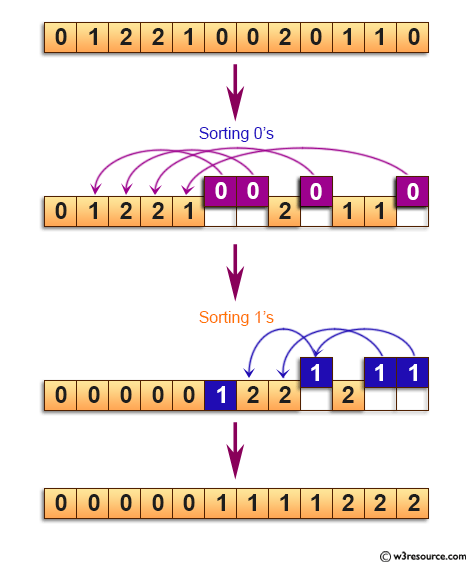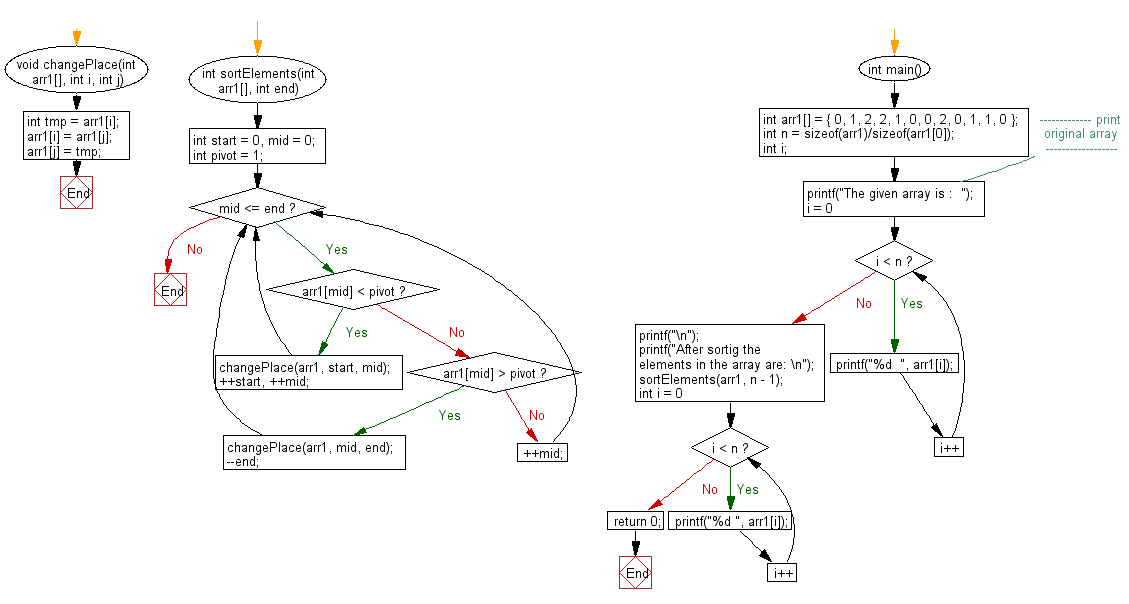﻿ C exercises: Sort an array of 0s, 1s and 2s - w3resource# C Exercises: Sort an array of 0s, 1s and 2s

## C Array: Exercise-54 with Solution

Write a program in C to sort an array of 0s, 1s and 2s.

Pictorial Presentation:Sample Solution:

C Code:

``````#include <stdio.h>
void changePlace(int arr1[], int i, int j)
{
int tmp = arr1[i];
arr1[i] = arr1[j];
arr1[j] = tmp;
}
int sortElements(int arr1[], int end)
{
int start = 0, mid = 0;
int pivot = 1;
while (mid <= end)
{
if (arr1[mid] < pivot)
{
changePlace(arr1, start, mid);
++start, ++mid;
}
else if (arr1[mid] > pivot)
{
changePlace(arr1, mid, end);
--end;
}
else
{
++mid;
}
}
}

int main()
{
int arr1[] = { 0, 1, 2, 2, 1, 0, 0, 2, 0, 1, 1, 0 };
int n = sizeof(arr1)/sizeof(arr1);
int i;
//------------- print original array ------------------
printf("The given array is :  ");
for(i = 0; i < n; i++)
{
printf("%d  ", arr1[i]);
}
printf("\n");
//------------------------------------------------------
printf("After sortig the elements in the array are: \n");
sortElements(arr1, n - 1);
for (int i = 0 ; i < n; i++)
{
printf("%d ", arr1[i]);
}
return 0;
}```
```

Sample Output:

```The given array is :  0  1  2  2  1  0  0  2  0  1  1  0
After sortig the elements in the array are:
0 0 0 0 0 1 1 1 1 2 2 2
```

Flowchart:C Programming Code Editor:

Improve this sample solution and post your code through Disqus.

What is the difficulty level of this exercise?

﻿